# Problema do caixeiro-viajante (Traveling Salesperson Problem)¶

Dado um conjunto de cidades, e as distâncias entre cada par de cidades, encontrar um tour das cidades com a distância mínima total. Um tour (passeio) significa que você começar em uma cidade, visitar qualquer outra cidade exatamente uma vez, e, em seguida, retornar à cidade de partida.

• Este é um conhecido problema intratável (intractable#Intractability)), o que significa que não existem soluções eficientes que trabalham para um grande número de cidades.
• Mas podemos criar um algoritmo ineficiente que funciona bem para um pequeno número de cita (cerca de uma dúzia) . Nós também podemos encontrar um tour quase - menor ao longo de milhares de cidades.
• Na verdade, o fato de que não há algoritmo eficiente é libertadora: significa que podemos utilizar um algoritmo muito simples, ineficiente e não me sinto muito mal com isso.
• Neste exercício, vamos olhar para alguns algoritmos para resolver o problema . A lição mais importante é a forma de pensar sobre como resolver um problema como este; os pormenores deste problema particular são menos importantes.

Vamos pensar primeiro sobre o vocabulário do problema: quais são os conceitos importantes e estamos certos de que entendemos bem o suficiente para implementar os conceitos (como dados ou funções)?

Nós vamos começar a considerar possíveis implementações , na linguagem de programação Python, mas não vai fazer escolhas definitivas ainda:

• Cidade: Para efeitos deste exercício, a cidade é "atômica", no sentido de que não precisa saber nada sobre os componentes ou atributos de uma cidade ; nós não precisamos saber o seu nome , população, ou qualquer outra coisa , a não ser que nós temos que saber o quão longe é a partir de outras cidades.
• Cidades: Vamos precisar para representar um conjunto de cidades ; o tipo de dados Set do Python pode ser apropriado para isso.
• Distância: Vamos precisar a distância entre duas cidades. Se A e B são cidades , isso poderia ser feito com uma função , a distance(A, B), ou com um dicionário, distance[A][B], ou uma matriz distance[A,B], se A e B são índices inteiros. A distância resultante será um número real (que Python chama um float).
• Tour: Um passeio é uma lista ordenada das cidades; Lista ou tupla tipos de dados do Python iria funcionar.
• Distância total: A distância total para um tour é a soma das distâncias de cidades adjacentes na turnê: total_distance(tour).

## Python and iPython Notebook: Preliminares¶

Primeiro importamos algumas bibliotecas que vamos a precisar.

In :
import matplotlib
import matplotlib.pyplot as plt
import random
import time
import itertools
%matplotlib inline


## Algoritmo 1: Testar tudos os caminhos (exact_TSP)¶

Aqui é o nosso primeiro algoritmo, que encontra a turnê com menor distância total, com uma abordagem simples:

Gerar todos os possíveis passeios das cidades, e escolher o mais curto (a turnê com a distância mínima total).

Podemos implementar esta como a função exact_TSP. Aqui está a filosofia de design, vamos utilizar:

Escrever código Python que espelha a descrição do algoritmo. Isso provavelmente vai exigir algumas funções auxiliares e estruturas de dados; simplesmente assumir que será capaz de defini-los, bem como, usando a mesma filosofia de design.

In :
def exact_TSP(cities):
"Generate all possible tours of the cities and choose the shortest one."
return shortest(alltours(cities))

def shortest(tours):
"Return the tour with the minimum total distance."
return min(tours, key=total_distance)


Nota 1: Ainda não definimos total_distance ou alltours.

Nota 2: No Python min(collection,key=function) acha o elemento x que è parte da collection asim que function(x) è minimizada.

## Representação dos Tours¶

• Um tour começa em uma cidade, e, em seguida, visita a cada uma das outras cidades em ordem, antes de finalmente retirning para o início.
• Uma representação natural do conjunto de cidades disponíveis é um set Python.
• Uma representação natural de um tour é uma sequência que é uma permutação do conjunto.

Abaixo listamos os seis possíveis passeios/permutações de um conjunto de três cidades. A tupla (1, 2, 3), por exemplo, representa um tour que começa em 1 cidade, move-se para 2, depois 3, e em seguida, retorna a 1 para terminar a turnê.

Nota 3: Eu pensei em usar (1, 2, 3, 1) como a representação de uma turnê que começa e termina na cidade 1, mas decidi que (1, 2, 3) é melhor porque não é redundante.)

In :
alltours = itertools.permutations # The permutation function is already defined in the itertools module

cities = {1, 2, 3}

list(alltours(cities))

Out:
[(1, 2, 3), (1, 3, 2), (2, 1, 3), (2, 3, 1), (3, 1, 2), (3, 2, 1)]

## Representing Cities and Distance¶

Agora, para a noção de distância. Definimos total_distance(tour) como a soma das distâncias entre cidades consecutivas na turnê; essa parte é mostrado abaixo e é fácil.

• truque Python: quando i for 0, então a distance(tour , tour [-1]) nos dá a distância "wrap-around" entre o primeiro eo último cidades, porque tour[-1] é o último elemento de tour).
In :


  File "<ipython-input-11-8042364d3a7e>", line 1
a = distance(alltours, alltours) for i in range(len(tour))
^
SyntaxError: invalid syntax

In :
def total_distance(tour):
"The total distance between each pair of consecutive cities in the tour."
return sum(distance(tour[i], tour[i-1]) for i in range(len(tour)))


Before we can define distance(A, B), the distance between two cities, we have to make a choice. In the fully general version of the TSP problem, the distance between two cities could be anything: it could be the amount of time it takes to travel between cities, the number of dollars it costs, or anything else. So the distances could be represented by a matrix distance[A][B], where any entry in the matrix could be any numeric value.

But we will make the choice not to allow that full generality, and instead restrict ourselves to Euclidean distance, the straight-line distance between points in a two-dimensional plane. So each city will be represented by a two-dimensional point: a pair of x and y coordinates. We will use the constructor function City, so that City(300, 0) creates a city with x-coordinate of 300 and y coordinate of 0. then distance(A, B) will be a function that uses the x and y coordinates to compute the distance between A and B.

How will we represent a two-dimensional point? Here are some choices, with their pros and cons:

• Tuple: A point (or city) is a two-tuple of (x, y) coordinates, for example, (300, 0). Pro: Very simple, easy to break a point down into components. Reasonably efficient. Con: doesn't distinguish points from other two-tuples. If p is a point, can't do p.x or p.y.

• class: Define City as a custom class with x and y fields. Pro: explicit, gives us p.x accessors. Con: less efficient because of the overhead of creating user-defined objects.

• complex: Python already has the two-dimensional point as a built-in numeric data type, but in a non-obvious way: as complex numbers, which inhabit the two-dimensional (real × complex) plane. We can make this use more explicit by defining "City = complex", meaning that we can construct the representation of a city using the same constructor that makes complex numbers. Pro: most efficient, because it uses a builtin type that is already a pair of numbers. The distance between two points is simple: the absolute value of their difference. Con: it may seem confusing to bring complex numbers into play; can't say p.x.

• subclass: Define "class Point(complex): pass", meaning that points are a subclass of complex numbers. Pro: All the pros of using complex directly, with the added protection of making it more explicit that these are treated as points, not as complex numbers. Con: less efficient than using complex directly; still can't do p.x or p.y.

• subclass with properties: Define "class Point(complex): x, y = property(lambda p: p.real), property(lambda p: p.imag)". Pro: All the pros of previous approach, and we can finally say p.x. Con: less efficient than using complex directly.

Any of these choices would work perfectly well; I chose to go with complex numbers:

In :
City = complex # Constructor for new cities, e.g. City(300, 400)

def distance(A, B):
"The distance between two points."
return abs(A - B)


Here's an example of computing the distance between two cities:

In :
A = City(300, 0)
B = City(0, 400)
distance(A, B)

Out:
500.0

Here's how to make a set of random cities:

In :
def Cities(n):
"Make a set of n cities, each with random coordinates."
return set(City(random.randrange(10, 890), random.randrange(10, 590)) for c in range(n))

# Let's make some standard sets of cities of various sizes.
# We'll set the random seed so that these sets are the same every time we run this notebook.
random.seed('seed')
cities8, cities10, cities100, cities1000 = Cities(8), Cities(10), Cities(100), Cities(1000)

cities8

Out:
{(598+518j),
(880+382j),
(567+157j),
(604+81j),
(783+309j),
(156+117j),
(100+211j),
(625+335j)}

Now we are ready to apply the exact_TSP function to find the minimal tour through the set of cities cities8:

In :
tour = exact_TSP(cities8)

print(tour)
print(total_distance(tour))

%timeit exact_TSP(cities8)

((604+81j), (567+157j), (625+335j), (783+309j), (880+382j), (598+518j), (100+211j), (156+117j))
2010.23045477
10 loops, best of 3: 180 ms per loop


## Algorithm 1.1: Try All Non-Redundant Tours¶

The permutation (1, 2, 3) represents the tour that goes from 1 to 2 to 3 and back to 1. You may have noticed that there aren't really six different tours of three cities: the cities 1, 2, and 3 form a triangle; any tour must connect the three points of the triangle; and there are really only two ways to do this: clockwise or counterclockwise. In general, with $n$ cities, there are $n!$ (that is, $n$ factorial) permutations, but only $(n-1)!$, tours that are distinct: the tours 123, 231, and 312 are three ways of representing the same tour.

So we can make our TSP program $n$ times faster by never considering redundant tours. Arbitrarily, we will say that all tours must start with the "first" city in the set of cities. We don't have to change the definition of TSP—just by making alltours return only nonredundant tours, the whole program gets faster.

(While we're at it, we'll make tours be represented as lists, rather than the tuples that are returned by permutations. It doesn't matter now, but later on we will want to represent partial tours, to which we will want to append cities one by one; that can only be done to lists, not tuples.)

In :
def alltours(cities):
"Return a list of tours, each a permutation of cities, but each one starting with the same city."
start = first(cities)
return [[start] + list(tour)
for tour in itertools.permutations(cities - {start})]

def first(collection):
"Start iterating over collection, and return the first element."
for x in collection: return x


We can verify that for 3 cities there are now 2 tours (not 6) and for 4 cities there are 6 tours (not 24).

In :
alltours({1, 2, 3})

Out:
[[1, 2, 3], [1, 3, 2]]
In :
alltours({1, 2, 3, 4})

Out:
[[1, 2, 3, 4],
[1, 2, 4, 3],
[1, 3, 2, 4],
[1, 3, 4, 2],
[1, 4, 2, 3],
[1, 4, 3, 2]]

We can also verify that calling exact_TSP(cities8) still works and gives the same tour with the same total distance. (But it is now about 8 times faster.)

In :
tour = exact_TSP(cities8)

print(tour)
print(total_distance(tour))
%timeit exact_TSP(cities8)

[(598+518j), (880+382j), (783+309j), (625+335j), (567+157j), (604+81j), (156+117j), (100+211j)]
2010.23045477
10 loops, best of 3: 24 ms per loop


## Plotting¶

Looking at a long list of numbers is not very enlightening; if we could plot a tour we'd understand it better. I define plot_tour(algorithm, cities) to apply a TSP algorithm to a set of cities, plot the resulting tour, and print information about the total distance of the tour and the time it took to find it. The plot will have each city as a blue circle, except the starting point will be a red square:

In :
def plot_tour(algorithm, cities):
"Apply a TSP algorithm to cities, and plot the resulting tour."
# Find the solution and time how long it takes
t0 = time.clock()
tour = algorithm(cities)
t1 = time.clock()
# Plot the tour as blue lines between blue circles, and the starting city as a red square.
plotline(list(tour) + [tour])
plotline([tour], 'rs')
plt.show()
print("{} city tour; total distance = {:.1f}; time = {:.3f} secs for {}".format(
len(tour), total_distance(tour), t1-t0, algorithm.__name__))

def plotline(points, style='bo-'):
"Plot a list of points (complex numbers) in the 2-D plane."
X, Y = XY(points)
plt.plot(X, Y, style)

def XY(points):
"Given a list of points, return two lists: X coordinates, and Y coordinates."
return [p.real for p in points], [p.imag for p in points]

plot_tour(exact_TSP, cities8)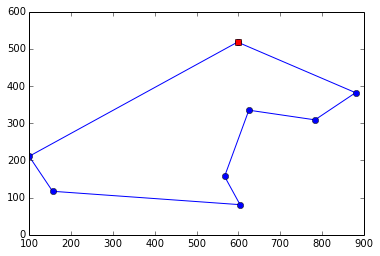8 city tour; total distance = 2010.2; time = 0.042 secs for exact_TSP


The plot looks much better than a list of coordinates! Now let's try a much harder 10-city tour:

In :
plot_tour(exact_TSP, cities10)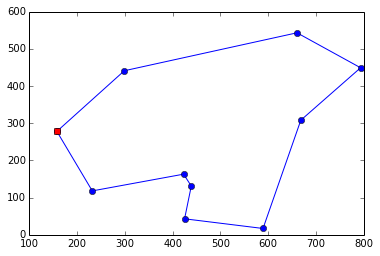10 city tour; total distance = 1906.9; time = 2.922 secs for exact_TSP


It takes about 3 seconds on my machine to solve this problem. In general, the function TSP looks at (n-1)! tours for an n-city problem, and each tour has $n$ cities, so the time for $n$ cities should be roughly proportional to n!. This means that the time grows rapidly with the number of cities; we'd need longer than the age of the Universe to run exact_TSP on just 24 cities:

n citiestime
103 secs
123 secs × 12 × 11 = 6.6 mins
146.6 mins × 13 × 14 = 20 hours
243 secs × 24! / 10! = 16 billion years

There must be a better way ...

# Approximate Algorithms¶

The general, exact Traveling Salesperson Problem is intractable; there is no efficient algorithm to find the tour with minimum total distance. But if we restrict ourselves to Euclidean distance and if we are willing to settle for a tour that is reasonably short but not the shortest, then the news is much better. We will consider several approximate algorithms, which find tours that are usually within 10 or 20% of the shortest possible and can handle thousands of cities in a few seconds.

## Algorithm 2: Greedy Nearest Neighbor (greedy_TSP)¶

Here is our first approximate algorithm:

Start at any city; at each step extend the tour by moving from the previous city to its nearest neighbor that has not yet been visited.

This is called a greedy algorithm, because it greedily takes what looks best in the short term (the nearest neighbor) even when that won't always be the best in the long term. To implement the algorithm I need to represent all the noun phrases in the English description: "start" (a city; arbitrarily the first city); "the tour" (a list of cities, initialy just the start city); "previous city" (the last element of tour, that is, tour[-]); "nearest neighbor" (a function that, when given a city, A, and a list of other cities, finds the one with minimal distance from A); and "not yet visited" (we will keep a set of unvisited cities; initially all cities but the start city are unvisited).

Once these are initialized, we repeatedly find the nearest unvisited neighbor, C, and add it to the tour and remove it from unvisited:

In :
def greedy_TSP(cities):
"At each step, visit the nearest neighbor that is still unvisited."
start = first(cities)
tour = [start]
unvisited = cities - {start}
while unvisited:
C = nearest_neighbor(tour[-1], unvisited)
tour.append(C)
unvisited.remove(C)

def nearest_neighbor(A, cities):
"Find the city in cities that is nearest to city A."
return min(cities, key=lambda x: distance(x, A))


(In Python, as in the formal mathematical theory of computability, lambda is the symbol for function, so "lambda x: distance(x, A)" means the function of x that computes the distance from x to the city A. The name lambda comes from the Greek letter λ.)

We can compare the fast approximate greedy_TSP algorithm to the slow exact_TSP algorithm on a small map, as shown below. (If you have this page in a IPython notebook you can repeatedly run the cell, and see how the algorithms compare. Cities(9) will return a different set of cities each time. I ran it 20 times, and only once did the greedy algorithm find the optimal solution, but half the time it was within 10% of optimal, and it was never more than 25% worse than optimal.)

In :
cities = Cities(9)
plot_tour(exact_TSP, cities)
%timeit greedy_TSP(cities)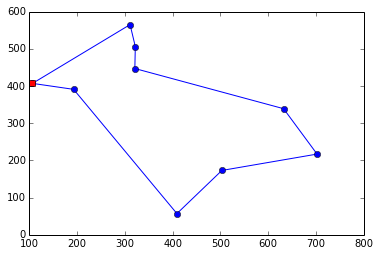9 city tour; total distance = 1685.3; time = 0.422 secs for exact_TSP
10000 loops, best of 3: 29.2 µs per loop

In [ ]:



But the key is that the greedy algorithm can quickly tackle problems that the optimal algorithm can't touch. Finding a tour of 100 or even 1000 cities takes less than a second:

In :
plot_tour(greedy_TSP, cities100)
plot_tour(greedy_TSP, cities1000)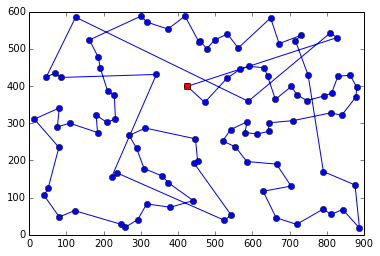100 city tour; total distance = 7532.7; time = 0.007 secs for greedy_TSP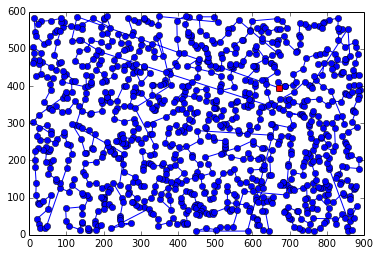999 city tour; total distance = 21169.0; time = 0.255 secs for greedy_TSP


Can we do better? Can we find shorter tours without taking an exponentially increasing amount of time? Our challenge is to combine the speed of the greedy algorithm with the precision of the exact algorithm.

We need an idea for how to get there ...

## Think of a Number from 1 to n!¶

Here's an idea: the exact_TSP algorithm is too slow for large n, because it considers all $n!$ tours. But the greedy_TSP algorithm considers exactly one tour—the tour that starts at an arbitrary city and then transitions to the nearest neighbor (repeatedly). Could we get a good compromise between execution speed and total tour distance if we considered more than 1 but less than $n!$ tours? What's a number between 1 and $n!$, and how could we use that to create a new algorithm?

## Algorithm 3: Greedy Nearest Neighbor from All Starting Points (all_greedy_TSP)¶

Let's think about how to improve the greedy algorithm. In the plot of the 100-city tour with greedy_TSP, we see that there are a few very-long edges between cities. These come about because we get to a certain point in the greedy construction of the tour where there are no close-by cities, and we have to jump far away. In a way, this just seems like bad luck—the way we flow from neighbor to neighbor just happens to leave a few very-long edges. Just as with buying lottery tickets, we can improve our luck by trying more often:

For each city, run the greedy algorithm with that city as the starting point, and choose the resulting tour with the shortest total distance.

So, with $n$ cities we run the greedy_TSP algorithm $n$ times, meaning the run time will be $n$ times longer, but the resulting tour will (in most cases) be shorter (and always at least as short as greedy_TSP). To implement all_greedy_TSP we just take the shortest tour over all starting cities. To do that requires a modification of greedy_TSP so that the starting city can be specified as an optional argument. Previously, greedy_TSP always used the first city as the strating point. We will make start an optional parameter; if it is unspecified, we default to the old behavior of using the first city.

In :
def all_greedy_TSP(cities):
"Try the greedy algorithm from each of the starting cities; return the shortest tour."
return shortest(greedy_TSP(cities, start=c) for c in cities)

# We will modify greedy_TSP to take an optional start city; otherwise it is unchanged.

def greedy_TSP(cities, start=None):
"At each step, visit the nearest neighbor that is still unvisited."
if start is None: start = first(cities)
tour = [start]
unvisited = cities - {start}
while unvisited:
C = nearest_neighbor(tour[-1], unvisited)
tour.append(C)
unvisited.remove(C)

# Compare greedy_TSP to all_greedy_TSP
plot_tour(greedy_TSP, cities100)
plot_tour(all_greedy_TSP, cities100)100 city tour; total distance = 7532.7; time = 0.007 secs for greedy_TSP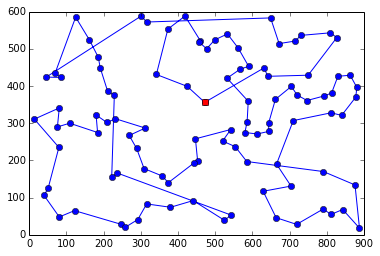100 city tour; total distance = 6802.5; time = 0.262 secs for all_greedy_TSP


We see that all_greedy_TSP does indeed take about $n=100$ times longer to run, but it yields a tour that is 8% shorter than greedy_TSP (for this particular 100-city map). For the 1000-city problem it would take about 1000 times longer. I didn't want to wait that long, so I didn't try. Instead let's look for other algorithms that better balance speed and shorter tours.

## Algorithm 4: Greedy Nearest Neighbor with Exact End (greedy_exact_end_TSP)¶

Of the several very-long edges between cities, it looks like most of them appear near the end of the tour. That makes sense: when there are only a few cities left, it is not surprising that the nearest one might be far away. So here's an idea:

Use the greedy algorithm for all but the last few cities (about 8), then use the exact algorithm over all possible ways to complete the tour.

This is another example of the "pick a number from 1 to $n!$" idea, this time picking $8!$ as the number of tours to consider. We'll define the function greedy_exact_end_TSP to take a parameter end_size to say how many cities at the end we will consider an exact solution for. The default is end_size=8. Suppose we are solving a 1000-city problem and have constructed a greedy tour through 992 cities, and now want to complete the final 8 cities. We will consider all 8! = 40,320 permutations of those cities. The simple way to do that is: generate all permutations of the 8 cities, and one at a time, append the 8 onto the 992 to yield a 1000-city tour, and calculate the total-distance for each such tour. Choose the shortest.

But that means we're computing total_distance of a 1000-city tour 40,320 times. It would be 100 times faster if we could just compute the total_distance of 10-city tours instead of 1000-city tours. Fortunately, we can do that. Since all 992 cities of the greedy part of the tour stay the same, their contribution to the total distance is the same for every permutation, except for the contribution of the first and last city out of the 992. So we can omit the 990 cities in the middle of the greedy part, and just evaluate the total_distance over a tour consisting of the first and last cities of the 992, followed by a permutation of the 8 remaining cities. Again, try them all and choose the shortest, and once we have the shortest, put the omitted cities back in.

In :
def greedy_exact_end_TSP(cities, start=None, end_size=8):
"""At each step, visit the nearest neighbor that is still unvisited until
there are k_end cities left; then choose the best of all possible endings."""
if start is None: start = first(cities)
tour = [start]
unvisited = cities - {start}
# Use greedy algorithm for all but the last end_size cities
while len(unvisited) > end_size:
C = nearest_neighbor(tour[-1], unvisited)
tour.append(C)
unvisited.remove(C)
# Consider all permutations of possible ends to the tour, and choose the best one.
# (But to make things faster, omit the middle of the tour.)
ends = map(list, itertools.permutations(unvisited))
best = shortest([tour, tour[-1]] + end for end in ends)

plot_tour(greedy_exact_end_TSP, cities100)
plot_tour(greedy_exact_end_TSP, cities1000)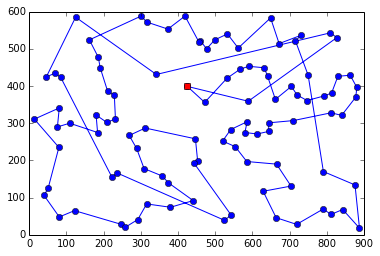100 city tour; total distance = 7258.3; time = 0.432 secs for greedy_exact_end_TSP999 city tour; total distance = 21169.0; time = 0.542 secs for greedy_exact_end_TSP


We see that greedy_exact_end_TSP is a bit disapointing on the 100-city map; it gives a longer tour than all_greedy_TSP and is no faster. But on the 1000-city map, it is faster than the expected run time of all_greedy_TSP, and yields a slightly shorter tour than greedy_TSP.

## Algorithm 5: Greedy Nearest Neighbor with Both Ends Search (greedy_bi_TSP)¶

Should we spend our computation time on different cities at the start of the tour (as in all_greedy_TSP) or on different permutations at the end of the tour (as in greedy_exact_end_TSP)? Why not do both?

In the function greedy_bi_TSP we combine an end_size parameter to say how many of the final cities to do exact search over, and a start_size parameter to say how many different starting cities to try. We won't necessarily try all starting cities, and the ones we do try will be sampled at random.

We try the algorithm with a default of 12 starting cities and 7 cities at the end; the results on both the 100-city and 1000-city maps are shorter than any other algorithm we have seen so far. That's encouraging, but with other choices of random starting points the results might be different, and on other maps the results might be different. I would want more convincing evidence before declaring this algorithm the champion.

In :
def greedy_bi_TSP(cities, start_size=12, end_size=6):
"At each step, visit the nearest neighbor that is still unvisited."
starts = random.sample(cities, min(len(cities), start_size))
return shortest(greedy_exact_end_TSP(cities, start, end_size)
for start in starts)

random.seed('bi')
plot_tour(greedy_bi_TSP, cities100)
plot_tour(greedy_bi_TSP, cities1000)

---------------------------------------------------------------------------
NameError                                 Traceback (most recent call last)
<ipython-input-33-e13437f759d7> in <module>()
6
7 random.seed('bi')
----> 8 plot_tour(greedy_bi_TSP, cities100)
9 plot_tour(greedy_bi_TSP, cities1000)

<ipython-input-25-5ed0b4aa8e48> in plot_tour(algorithm, cities)
3     # Find the solution and time how long it takes
4     t0 = time.clock()
----> 5     tour = algorithm(cities)
6     t1 = time.clock()
7     # Plot the tour as blue lines between blue circles, and the starting city as a red square.

<ipython-input-33-e13437f759d7> in greedy_bi_TSP(cities, start_size, end_size)
3     starts = random.sample(cities, min(len(cities), start_size))
4     return shortest(greedy_exact_end_TSP(cities, start, end_size)
----> 5                     for start in starts)
6
7 random.seed('bi')

<ipython-input-2-f7d5f94fb837> in shortest(tours)
5 def shortest(tours):
6     "Return the tour with the minimum total distance."
----> 7     return min(tours, key=total_distance)

<ipython-input-33-e13437f759d7> in <genexpr>((start,))
3     starts = random.sample(cities, min(len(cities), start_size))
4     return shortest(greedy_exact_end_TSP(cities, start, end_size)
----> 5                     for start in starts)
6
7 random.seed('bi')

NameError: global name 'greedy_exact_end_TSP' is not defined

## Benchmarking Algorithms¶

If we only consider one or two maps we can't be confident which algorithm is best. It would be better to average over, say, 50 or 100 random maps at a time and see a summary of the total distances produced by different algorithms on these maps. We'll define the compare_algorithms function to take a list of TSP algorithms and a list of maps, and apply each algorithm to all the maps. For each algorithm we collect all the total distances on all the maps, and then sort the distances to get a monitonically increasing curve. We can then compare the curves: the lower the curve, the better. The chart legend also gives the total time in seconds and the average tour length for each algorithm.

Here is the code and a comparison plot:

In :
def compare_algorithms(algorithms, maps):
"Apply each algorithm to each map and plot results."
for algorithm in algorithms:
t0 = time.clock()
results = [total_distance(algorithm(m)) for m in maps]
t1 = time.clock()
avg = sum(results) / len(results)
label = '{:.0f}; {:.1f}s: {}'.format(avg, t1-t0, algorithm.__name__)
plt.plot(sorted(results), label=label)
plt.legend(loc=2)
plt.show()
print('{} x {}-city maps'.format(len(maps), len(maps)))

def Maps(M, N):
"Return a list of M maps, each consisting of a set of N cities."
return [Cities(N) for m in range(M)]

compare_algorithms([greedy_TSP, greedy_exact_end_TSP, all_greedy_TSP], Maps(100, 50))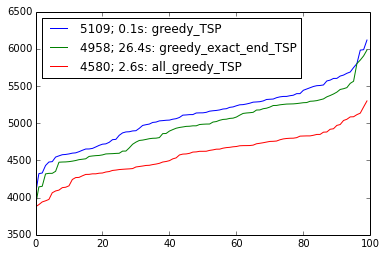100 x 50-city maps


We see that greedy_TSP (the blue line) is the fastest of the three algorithms, but gives the longest tours (as expected). greedy_exact_end is the slowest, by far, and only a little bit shorter than greedy_TSP. The winner looks like all_greedy_TSP; significantly shorter tours with not-too-long runtimes. This suggests that the multiple starting points are helping more than the permutation of ending cities, but let's explore the tradeoff by considering multiple points along the way:

In :
def bi_10_6(cities):  return greedy_bi_TSP(cities,  10, 6)
def bi_20_5(cities):  return greedy_bi_TSP(cities,  20, 5)
def bi_40_4(cities):  return greedy_bi_TSP(cities,  40, 4)
def bi_80_2(cities):  return greedy_bi_TSP(cities,  80, 2)
def bi_160_1(cities): return greedy_bi_TSP(cities, 160, 1)

algorithms = [bi_10_6, bi_20_5, bi_40_4, bi_80_2, bi_160_1]

In :
compare_algorithms(algorithms, Maps(100, 50))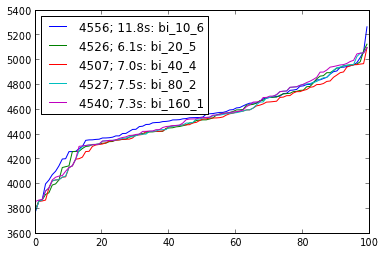100 x 50-city maps


We see that for 50-city maps all the parameter choices perform similarly. How about with 100-city maps?

In :
compare_algorithms(algorithms, Maps(50, 100))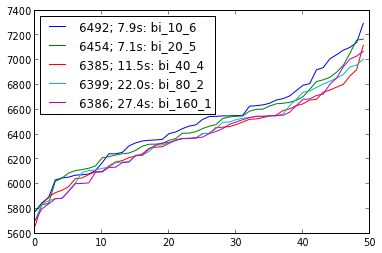50 x 100-city maps


Again, there is no clear winner. Let's try 160-city maps:

In :
compare_algorithms(algorithms, Maps(25, 160))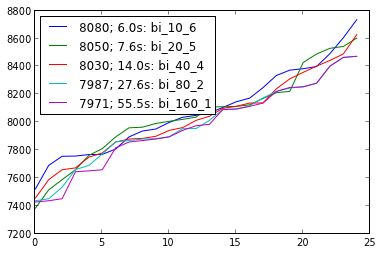25 x 160-city maps


We're starting to see a bit of separation: bi_160_1, which tries all starting cities and only one permutation at the end, is the best, but still not by a wide margin.

Now we could gather more statistics, and we could do a significance test to quantify the probability that the winning algorithm really is the best, or just benefitted from lucky random variation. Doing that kind of test is a useful exercise, but I feel like we should explore other completely different algorithms before spending times splitting hairs over the best parameters to greedy_bi_TSP.

We can do a postmortem analysis where we examine hat went wrong with the greedy algorithm and try to come up with ways to improve.

## The Trouble with Greedy: Outliers¶

In the 20-city map below, we give an illustrative example of where greedy algorithms go wrong. We have 4 cities scattered around the outskirts of an inner square of 16 cities. The issue is that a greedy algorithm that starts anywhere in the inner square will traverse the whole square, ignoring the outlying points, and then at the end we will be left with long paths to the outliers.

In :
outliers_list = [City(2, 2),  City(2, 3),  City(2, 4),  City(2, 5),  City(2, 6),
City(3, 6),  City(4, 6),  City(5, 6),  City(6, 6),
City(6, 5),  City(6, 4),  City(6, 3),  City(6, 2),
City(5, 2),  City(4, 2),  City(3, 2),
City(1, 6.8),  City(7.8, 6.4),  City(7, 1.2),  City(0.2, 2.8)]

plotline(outliers_list, 'bo')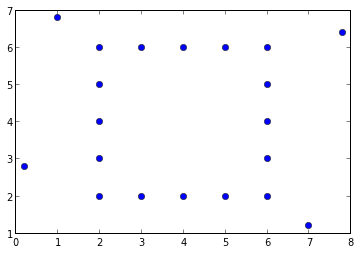For example, in the plot below, the greedy_TSP algorithm tour starts at the red point, moves clockwise around the inner square, and then picks up the four outlying points in counter-clockwise order, with four very long edges.

In :
outliers = set(outliers_list)

plot_tour(greedy_TSP, outliers)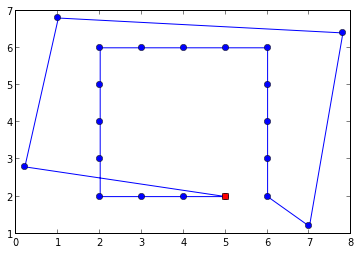20 city tour; total distance = 37.3; time = 0.000 secs for greedy_TSP


all_greedy_TSP has the same problem:

In :
plot_tour(all_greedy_TSP, outliers)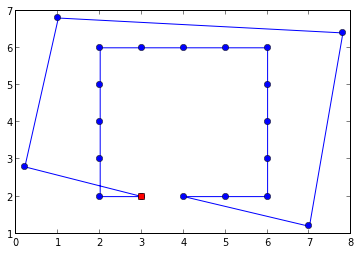20 city tour; total distance = 37.2; time = 0.006 secs for all_greedy_TSP


The algorithms that search over permutations of ending cities (greedy_exact_end_TSP and greedy_bi_TSP) do somewhat better: an end-size of 8 is enough to pick up two of the four outliers in a reasonable postion. But not all four outliers.

In :
plot_tour(greedy_exact_end_TSP, outliers)
plot_tour(greedy_bi_TSP, outliers)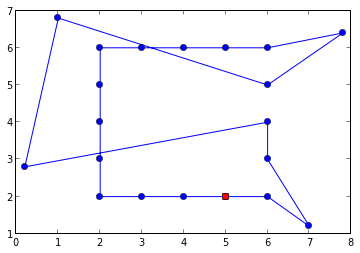20 city tour; total distance = 35.8; time = 0.655 secs for greedy_exact_end_TSP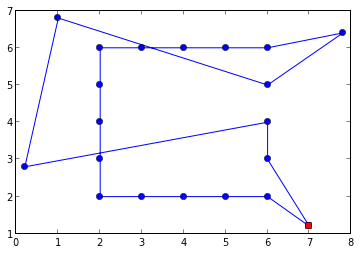20 city tour; total distance = 35.8; time = 0.123 secs for greedy_bi_TSP


Let's try to understand what went wrong. First we'll create some more tools to better draw diagrams.

In :
def plot_lines(points, labels, *args):
"""Takes a list of points and a (possibly empty) list of labels for them,
followed by any number of args which can be either a string, indicating a new line style, like 'bo-',
or a list of indexes into points, like [0, 1, 2, 3], which indicates a line through those points."""
plot_points(points, labels)
style = 'bo-'
for arg in args:
if isinstance(arg, str):
style = arg
else: # arg is a list of indexes into points, forming a line
line = [points[i] for i in arg]
X, Y = XY(line)
plt.plot(X, Y, style)
plt.show()

def plot_points(points, labels=(), style='bo'):
"Plot individual points (and maybe labels for them) without any lines."
plot_labels(points, labels)
for p in points:
plt.plot([p.real], [p.imag], style)

def plot_labels(cities, labels):
"If any labels are supplied, plot them."
for (c, L) in zip(cities, labels):
plt.text(c.real, c.imag, '  '+str(L))


In the diagram below, imagine we are running some variation of a greedy algorithm, and it has created a partial tour from city 0 to city 4. Now there is a choice. City 5 is the nearest neighbor. But if we don't take city 16 at this point, we will have to pay a higher price sometime later to pick up city 16. This is an example of when it doesn't pay to be greedy in the short run—we need to think about the future consequences of not selecting 16 now.

In :
plot_lines(outliers_list, range(20), range(0, 5), 'ro--', [4, 16], 'bo--', [4, 5])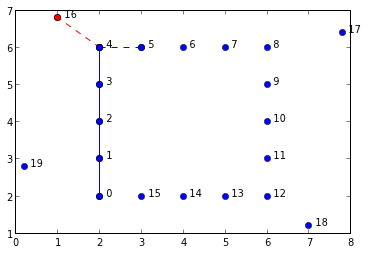## Algorithm 6: Nearest Neighbors of a city and to a city (double_greedy_TSP)¶

How could we fix the issue? Let's arrange to not skip a city when it is its time. That is, even if outlying city C (like city 16 in the diagram above) does not happen to be the nearest neighbor to the tour's endpoint (city 4 in the diagram above), if C is not near to any other city, then probably now is a good time to add it to the tour. That is, I should add C to the tour if either C is the nearest neighbor of the tour's endpoint, or if C's nearest neighbor is the tour's endpoint. We call this algorithm double_greedy_TSP. For efficiency, it precomputes the nearest neighbor of each city and stores it the dict NN. It tracks the tour and the unvisited cities, just like greedy_TSP. On each iteration, it does this: call the last city in the tour A, and its nearest neighbor B. Then form the set Ds of all cities for whom A is the nearest neighbor. If there are any, make the chosen city, C be the closest member of Ds; if there aren't any, make C be the nearest neighbor, B. Add C to the tour and remove it from unvisited.

In :
def double_greedy_TSP(cities, start=None):
"""At each step, call the last city in the tour A, and consider the
nearest neighbor B, and also any city D that has A as nearest neighbor."""
NN = {C: nearest_neighbor(C, cities - {C}) for C in cities}
if start is None: start = first(cities)
tour = [start]
unvisited = cities - {start}
while unvisited:
A = tour[-1]
B = NN[A] if NN[A] in unvisited else nearest_neighbor(A, unvisited)
Ds = [D for D in unvisited if NN[D] is A and D is not B]
C = (min(Ds, key=lambda D: distance(D, A))) if Ds else B
tour.append(C)
unvisited.remove(C)

plot_tour(double_greedy_TSP, outliers)
compare_algorithms([greedy_TSP, double_greedy_TSP, all_greedy_TSP], Maps(100, 50))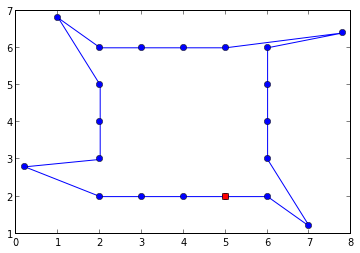20 city tour; total distance = 27.1; time = 0.001 secs for double_greedy_TSP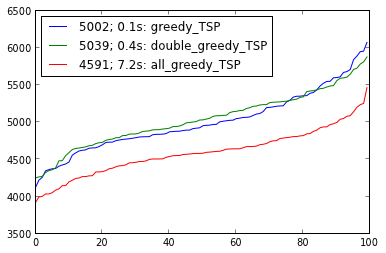100 x 50-city maps


We see that double_greedy_TSP completely solves the outliers map. Unfortunately, it fails to turn in a distinguished performance on a collection of 50-city maps, performing roughly the same as the simple greedy_TSP (but slower). It seems that it is not always a good idea to pick up an outlier. So what can we do? Let's take a moment to reflect on what we have done so far, and what we might yet do.

## Overall Strategies¶

• Better choices: figure out when it is a good idea to extend the tour to a non-nearest-neighbor, and when it is not.

• More choices: consider both including and not including a non-nearest neighbor. We will have multiple possible partial tours under consideration at any time.

• Recovery from bad choices: make one choice, but allow for the possibility of making changes. We will quickly build one single complete tour, and then will consider alterations to the tour.

SO far we've seen 6 algorithms, but only two basic strategies:

• Exact algorithms: Try all tours and choose the best.

• Greedy algorithms: Start with a partial tour (initially one city) and keep extending it by adding a near neighbor.

Now we'll introduce a third basic strategy:

• Improvement algorithms: Use an old algorithm to produce a tour, then improve the tour by trying many small alterations, and keeping those that make the tour shorter.

That's the basic idea, but there are many details to work out. First we'll look at some ways tours could be improved.

In general, in situations like this, there are three choices:

## Improving Tours by Reversing Segments¶

We'll define a segment as a subsequence of a tour: a sequence of consecutive cities within a tour. One way we could try to improve a tour is by reversing a segment. We'll look at an example, but first we'll need a tool for drawing segments. We'll call it plot_lines, and it takes the following arguments: first, a list of cities, second a sequence of labels for the cities (or None), and then any number of pairs of arguments that describe how to draw segments—first a list of indexes into the list of cities, and second a line style (in the format expected by the plot function).

In :
cross = [9+3j, 3+10j, 2+16j, 3+21j, 9+28j, 26+3j, 32+10j, 33+16j, 32+21j, 26+28j]

plot_lines(cross, range(10), range(-1,10), 'bo-')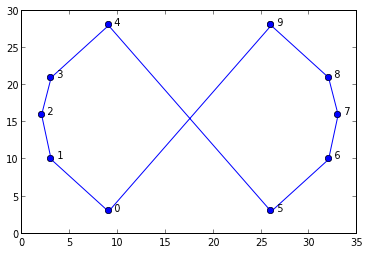The tour above is clearly not an optimal tour. We could improve it by "uncrossing" the lines. We can think of uncrossing the lines as reversing a segment. The tour as it stands is [0, 1, 2, 3, 4, 5, 6, 7, 8, 9]. If we reverse the segment [5, 6, 7, 8, 9], we get the tour [0, 1, 2, 3, 4, 9, 8, 7, 6, 5], which is the optimal tour. In the diagram below, reversing [5, 6, 7, 8, 9] is equivalent to deleting the dotted red lines and adding the dotted blue lines. We know that the reversal is in fact an improvement if the sum of the blue dotted lines is less than the sum of the red dotted lines.

In :
plot_lines(cross, range(10), 'bo-', range(5), range(5, 10), 'bo--', (4, 9), (0, 5),
'r--', (4, 5), (0, 9))

plot_points(outliers_list, range(20))
plot_lines(outliers_list, (), range(16), 'bo-')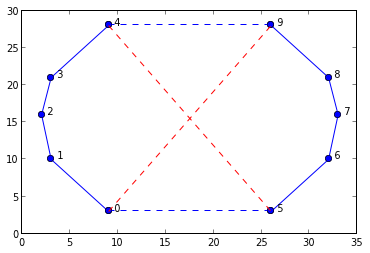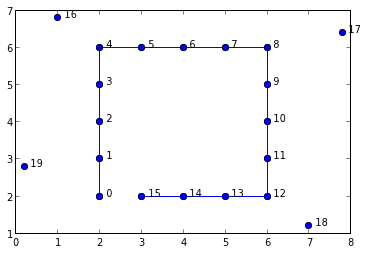Note that if distances are Euclidean, then any time there is a pair of crossed lines, the tour can be improved by uncrossing them.

Also note the general idea of an improvement algorithm: to allow a base algorithm to make mistakes, but to avoid suffering from those mistakes. We can use a greedy algorithm as a base algorithm, and it can make mistakes (like putting an outlier in the wrong place, or crossing lines) and we can still recover from those mistakes. Why arrange things with two parts to the algorithm, a greedy part followed by an improvement part? Because some decisions can be difficult to make when a partial tour is in the process of being created, but can be easy to make after the tour is completed. It is always wrong to cross lines, but when we are constructing a tour and laying down the first line, we don't know that it will eventually become part of a crossed pair. But after the tour is completed, we do know.

## Algorithm 7: Iterative Improvement (improved_greedy)¶

That leaves a lot of details to work out. We will:

• Try multiple starting cities, find a tour for each one, and improve each tour.
• Base the improvements on the result of greedy_exact_end_TSP.
• Try reversing each 2- or 3-city long segment
• Try moving each city to a better position in the tour
• Randomly try moving or reversing segments of different sizes
• When a large number of tries in a row fails to improve things, stop trying
In :
def improved_greedy(cities, start_size=10, end_size=4, num_fails=None):
"Improve the result of greedy_TSP from each starting point."
starts = cities if start_size >= len(cities) else random.sample(cities, start_size)
return shortest(improve(greedy_exact_end_TSP(cities, start, end_size), num_fails)
for start in starts)

RNG = random.randrange

def improve(tour, num_fails=None):
"Improve the tour by moving or reversing segments (tour[i:j])."
N = len(tour)
num_fails = num_fails or 4*N

# Try moving each individual city to a better spot
for i in range(N):
maybe_move_city(tour, i)

# Try reversing each 2- and 3-long segments
for i in range(N-2):
maybe_reverse_segment(tour, i, i+2)
for i in range(N-3):
maybe_reverse_segment(tour, i, i+3)

# Randomly pick segment tour[i:j], try to reverse or move it.
# If Ntries in a row fail to improve, then give up.
consecutive_fails = 0
while consecutive_fails < Nfails:
i = RNG(N-2)
j = RNG(i+2, N)
better = maybe_reverse_segment(tour, i, j) or maybe_move_somewhere(tour, i, j, cities)
consecutive_fails = 0 if better else consecutive_fails + 1

def maybe_reverse_segment(tour, i, j):
"If reversing tour[i:j] helps, then do it. Return True if it helps"
A, B, C, D = tour[i-1], tour[i], tour[j-1], tour[j]
# Are edges added less than edges taken away?
if distance(A, C) + distance(B, D) < distance(A, B) + distance(C, D):
tour[i:j] = reversed(tour[i:j])
return True

def maybe_move_cities(tour):
"Try to relocate each city to the best spot in the tour (closest to neighbors)."
N = len(tour)
positions = range(N)
dummy = object()
for i in positions:
A, B, C = tour[i-1], tour[i], tour[(i+1)%N]
# Try to move city B to the best place it could go: the minimum detour distance
j = min(positions, key=lambda j: detour(B, tour[j], tour[j-1]))
D, E = tour[j-1], tour[j]
if detour(B, D, E) < detour(B, A, C):
tour[i], tour[j:j] = dummy, [B]
tour.remove(dummy)

def detour(B, A, C):
"In going A-B-C, how much of a detour is this over just A-C?"
return distance(A, B) + distance(B, C) - distance(A, C)

#plot_tour(all_greedy_TSP, cities100)
plot_tour(improved_greedy, cities100)
#plot_tour(improved_greedy, tricky)

---------------------------------------------------------------------------
NameError                                 Traceback (most recent call last)
<ipython-input-1-e0215cbd61b5> in <module>()
5                     for start in starts)
6
----> 7 RNG = random.randrange
8
9 def improve(tour, num_fails=None):

NameError: name 'random' is not defined

## Algorithm 8: Google It, and do Minimum Spanning Tree (MTS_TSP)¶

Working with the greedy nearest neighbor algorithm may have given you some ideas for other algorithms (I know it did for me, and we'll get to them in a bit). But if you don't have an idea, you can always Google it, in which case you'll quickly find this algorithm:

Construct a Minimum Spanning Tree, then walk the tree in pre-order, omitting repeated nodes. That will give you a tour that is guaranteed to be no more than twice as long as the minimal tour.

What does all this jargon mean? We will first explain what a minimum spanning tree is, and then how to walk it in pre-order, then why you might want to do that. First a definition:

Given a set of vertexes (e.g. cities), a spanning tree is a tree that connects all the vertexes together. A tree is a collection of vertexes and edges such that there is exctly one path between any two vertexes: there are no loops, and no disconnected parts. A minimum spanning tree is a spanning tree such that the sum of the edge distances is as small or smaller than any other spanning tree.

Now an algorithm for finding a minimum spanning tree:

Consider all the edges (we'll represent an edge as a pair (A, B) of cities) and sort the edges, shortest first. Initialize a tree to be a single city (we'll arbitrarily shoose the first) with no edges coming out of the city. Now repeat the following until the tree contains all the cities: find the shortest edge that links a city (A) that is in the tree to a city (B) that is not yet in the tree, and add B to the list of A's links in the tree.

(Note we are using "link" and "edge" as synonyms (whichever sounds better at the time)). This is called Prim's algorithmHere's the code:

In [ ]:
def MST(cities):
"""Given a set of cities, build a minimum spanning tree: a dict of the form {parent: [child...]},
where parent and children are cities, and the root of the tree is first(cities)."""
N = len(cities)
edges = shortest_first([(A, B) for A in cities for B in cities if A is not B])
tree = {first(cities): []} # the first city is the root of the tree.
while len(tree) < N:
(A, B) = first((A, B) for (A, B) in edges if (A in tree) and (B not in tree))
tree[A].append(B)
tree[B] = []
return tree

def shortest_first(edges):
"Sort a list of edges so that shortest come first."
edges.sort(key=lambda (A, B): distance(A, B))
return edges

from collections import defaultdict

def MSTk(cities):
"""Go through edges, shortest first.  Always join two closest segments."""
edges = shortest_first([(A, B) for A in cities for B in cities if abs(A) < abs(B)])
tree = defaultdict(list)
subgraphs = {c: [c] for c in cities}
for (A, B) in edges:
if subgraphs[A] is not subgraphs[B]:
join_subgraphs(subgraphs, A, B)
tree[A].append(B)
tree[B].append(A)
if len(tree) == len(cities):
return tree

def join_subgraphs(subgraphs, A, B):
"Copy B's subgraph into A, and update."
As, Bs = subgraphs[A], subgraphs[B]
As.extend(Bs)
for c in Bs:
subgraphs[c] = As

plot_tree(MST(cities100))
plot_tree(MSTk(cities100))


How do we know this algorithm produces a minimum spanning tree? First, it is a tree because all the cities in the tree are connected (we start with just one, and every time we add a city, it is connected to one in the tree) and there are no loops (we add only one link to a city -- after that, it is in the tree, so we never add another link to it). Second, it is a spanning tree because it contains all the cities, and third, it is a minimum spanning tree because each city was added with the shortest possible edge. Suppose this algorithm produces the tree T. For another putative spanning tree to be shorter, it would have to contain at least one city C with an edge into C that is shorter than any edge into C in T.

In [ ]:
def plot_tree(tree):
"Given a tree of {parent: [child...]}, plot the lines between points."
for P in tree:
for C in tree[P]:
plotline([P, C], 'ko-')
plt.show()
print('{} node Minimum Spanning Tree of length: {:.1f}'.format(
len(tree), sum(distance(P, C) for P in tree for C in tree[P])))

mst = MST(cities100)
plot_tree(mst)

R = random.randrange

def Grid(w, h): return set(City(x+R(2,7)/10., y+R(2,7)/10.) for x in range(w) for y in range(h))
grid = Grid(15, 10)
plot_tree(MST(cities10))
plot_tree(MST(grid))
plot_tour(greedy_TSP, grid)
plot_tour(MST_TSP, grid)
plot_tour(improved_greedy, grid)

In [ ]:
def MST_TSP(cities):
"Create a minimum spanning tree and walk it in pre-order, omitting duplicates."
return preorder_uniq(MST(cities), first(cities), [])

def preorder_uniq(tree, node, result):
"Traverse tree in pre-order, starting at node, omitting repeated nodes."
# Accumulate results in the 'result' parameter, which should start with an empty list
if node not in result:
result.append(node)
for child in tree[node]:
preorder_uniq(tree, child, result)
return result

plot_tour(MST_TSP, cities100)
plot_tour(greedy_TSP, cities100)


## Algorithm 9: Joining Partial Tour Segments (greedy_join_TSP)¶

Can we do better than greedy_TSP without paying the time penalty of all_greedy_TSP? One idea is that instead of keeping one partial tour and always adding on to the end, we can instead keep multiple smaller tour segments: a segment is a list of 1 or more cities, in order, that will eventually fit into the final tour. Then the algorithm is:

Start with each city defining its own 1-city segment. At each step, join together the two segments that are closest to each other. Repeat until only one segment is left.

By closest, we mean the distance from one of the two ends of one segment to one of the ends of the other. That means that segments can join at either end, so a segment does not have a direction, but it does have an ordering. For example, if we have the two segments ABC and DE, we can join them 4 ways: ABC+DE, ABC+ED, CBA+DE, or CBA+ED. Note that ABCDE and EDCBA are considered the same segment. We also keep a dictionary of segments: segements[C] gives the segment that contains city C.

The way we implement this is to keep a list of edges in the form (A, B), where A and B are cities, and where the list is sorted so that shorter edges come first. Then we work through the list of edges, seeing if an edge can be used to join two segments.

Suppose we have the segments ABC and DE. If the next shortest edge is (A, C), then join_segments does nothing, because A and C are already in the same segment. If the next edge is (B, E) then again join_segments does nothing, because B is in the middle of a segment; it already has an incoming and outcoming edge, and can't be joined to something else. But if (A, D) is next, then we can join the segments: we reverse ABC to get CBA and then add DE to the end of CBA to form CBADE. (And we update segments[D] and segments[E] to be this joined segment.) We continue the joining process until there is one segment that covers all the cities.

Below we see the code, and the tour for the same 100 cities as shown above. Notice that on this particular map, greedy_join_TSP is 20 times faster than all_greedy_TSP and produces a tour that is within 1% in length.

In [ ]:
def greedy_join_TSP(cities):
"""Go through edges, shortest first.  Always join two closest segments."""
edges = shortest_first([(A, B) for A in cities for B in cities if abs(A) < abs(B)])
segments = {c: [c] for c in cities}
for (A, B) in edges:
join_segments_with(segments, A, B)
if len(segments[A]) == len(cities):
return segments[A]

def join_segments_with(segments, A, B):
"If the A-B edge can be used to join B's segment onto A's, then do it."
As, Bs = segments[A], segments[B]
# if they are not already joined and if A and B are at the ends of their segments
if As is not Bs and A in (As,As[-1]) and B in (Bs,Bs[-1]):
# Make As == [..., A] and Bs == [B, ...]
if As is A: As.reverse()
if Bs[-1] is B: Bs.reverse()
# Move all the cities in Bs over into As
As += Bs
for c in Bs:
segments[c] = As
return As

plot_tour(greedy_join_TSP, cities100)

In [ ]:
def greedy_join_TSP(cities, plot_sizes=()):
"""Go through edges, shortest first.  Always join two closest segments."""
edges = shortest_first([(A, B) for A in cities for B in cities if abs(A) < abs(B)])
segments = {c: [c] for c in cities}
for (A, B) in edges:
if join_segments(segments, A, B):
S = distinct_segments(segments)
if len(S) in plot_sizes:
map(plotline, S); plt.show()
print('{} cities with {} segments'.format(len(cities), len(S)))
if len(segments[A]) == len(cities):
return segments[A]
print map(len, segments.values())

def distinct_segments(segments):
return set(map(tuple, segments.values()))

greedy_join_TSP(cities100, {20, 10, 5})
plot_tour(greedy_join_TSP, cities100)


## Algorithm 10: Ensemble of Other Algorithms (ensemble_TSP)¶

Running out of ideas for algorithms? Don't have one algorithm that always out-performs all the others? Here's an idea: steal all the other ideas! The function ensemble_TSP combines the other algorithms we have defined in such a way that it is guaranteed to be best (except that it will be slower than running a single algorithm). If there are 10 or fewer cities, it finds the optimal solution with TSP. Otherwise, it tries each of greedy_join_TSP, double_greedy_TSP, and improved_greedy, then applies improve to each of the results, and returns the shortest tour. (It doesn't try greedy_TSP nor all_greedy_TSP, because they are subsumed by improved_greedy,) When compared to improved_greedy, we see ensemble_TSP is usually (but not always) better (and can never be worse).

In [ ]:
def ensemble_TSP(cities, algorithms={greedy_join_TSP, double_greedy_TSP}):
# jQuery17104754353954922408_1370288511049? MST_TSP, improved_greedy
"Apply all the algorithms and pick the shortest resulting tour."
if len(cities) <= 10:
return TSP(cities)
else:
return shortest(improve(tsp(cities)) for tsp in algorithms)

#plot_tour(improved_greedy, cities100)
plot_tour(ensemble_TSP, cities100)


We see that of the fast algorithms, greedy_TSP is twice as fast as the others, but greedy_join_TSP produces about 5% shorter tours.

Among the slower algorithms, ensemble_TSP and improved_greedy are similar, both producing tours about 10% to 15% shorter (but 80 times slower) than greedy_join_TSP. Meanwhile, all_greedy_TSP is intermediate in tour length and speed. (Note: unfortunately, greedy_join_TSP is red in the top graph and blue in the bottom; my aplogies if this causes confusion.)

We don't know what the optimal solutions are for those 50-city maps (we can't run TSP on them; it would take forever), but we can do a comparison on smaller (9-city) maps. It looks like ensemble_TSP is exactly identical to the optimal TSP in the tours it produces, and it runs at similar speed. greedy_join_TSP runs over 1000 times faster, but produces tours that are 5% to 10% longer.

In [ ]:
compare_algorithms([greedy_join_TSP, ensemble_TSP, TSP], Maps(100, 9))

In [ ]:
points = [(3+10j),(2+16j),(3+21j),(9+28j),(12+6j),(16+28j),(22+21j),(23+16j),(22+10j),(16+3j),(9+3j)]

plot_lines(points, None, range(11)+, 'bo-')

In [ ]:
plot_lines(points, None, (10, 0, 1, 2, 3), 'bo-', (5, 6, 7, 8, 9), 'bo-',
(3, 4, 5), 'r--', (9, 10), 'r--',
(10, 4, 9), 'bo--', (3, 5), 'bo--')

In :
def parse_city(line):
"Parse a line of data, as in http://www.realestate3d.com/gps/latlong.htm , and return a (City, cityname, state) triplet."
code, lat, long, name = line.split(None, 3)
cityname, state = name.split(',')
return City(-float(long), float(lat)), cityname, state

UScityData = map(parse_city, """
[TCL]  33.23   87.62  Tuscaloosa,AL
[PHX]  33.43  112.02  Phoenix,AZ
[PGA]  36.93  111.45  Page,AZ
[TUS]  32.12  110.93  Tucson,AZ
[LIT]  35.22   92.38  Little Rock,AR
[SFO]  37.62  122.38  San Francisco,CA
[LAX]  33.93  118.40  Los Angeles,CA
[SAC]  38.52  121.50  Sacramento,CA
[SAN]  32.73  117.17  San Diego,CA
[SBP]  35.23  120.65  San Luis Obi,CA
[EKA]  41.33  124.28  Eureka,CA
[SJC]  37.37  121.92  San Jose,CA
[DEN]  39.75  104.87  Denver,CO
[DRO]  37.15  107.75  Durango,CO
[HVN]  41.27   72.88  New Haven,CT
[DOV]  39.13   75.47  Dover,DE
[DCA]  38.85   77.04  Washington/Natl,DC
[MIA]  25.82   80.28  Miami Intl,FL
[TPA]  27.97   82.53  Tampa Intl,FL
[JAX]  30.50   81.70  Jacksonville,FL
[TLH]  30.38   84.37  Tallahassee,FL
[ATL]  33.65   84.42  Atlanta,GA
[BOI]  43.57  116.22  Boise,ID
[CHI]  41.90   87.65  Chicago,IL
[IND]  39.73   86.27  Indianapolis,IN
[DSM]  41.53   93.65  Des Moines,IA
[ICT]  37.65   97.43  Wichita,KS
[LEX]  38.05   85.00  Lexington,KY
[NEW]  30.03   90.03  New Orleans,LA
[BOS]  42.37   71.03  Boston,MA
[PWM]  43.65   70.32  Portland,ME
[BGR]  44.80   68.82  Bangor,ME
[DET]  42.42   83.02  Detroit,MI
[STC]  45.55   94.07  St Cloud,MN
[DLH]  46.83   92.18  Duluth,MN
[STL]  38.75   90.37  St Louis,MO
[JAN]  32.32   90.08  Jackson,MS
[BIL]  45.80  108.53  Billings,MT
[BTM]  45.95  112.50  Butte,MT
[RDU]  35.87   78.78  Raleigh-Durh,NC
[INT]  36.13   80.23  Winston-Salem,NC
[OMA]  41.30   95.90  Omaha/Eppley,NE
[LAS]  36.08  115.17  Las Vegas,NV
[EWR]  40.70   74.17  Newark Intl,NJ
[ABQ]  35.05  106.60  Albuquerque,NM
[SAF]  35.62  106.08  Santa Fe,NM
[LRU]  32.30  106.77  Las Cruces,NM
[NYC]  40.77   73.98  New York,NY
[BUF]  42.93   78.73  Buffalo,NY
[ALB]  42.75   73.80  Albany,NY
[FAR]  46.90   96.80  Fargo,ND
[BIS]  46.77  100.75  Bismarck,ND
[CVG]  39.05   84.67  Cincinnati,OH
[CLE]  41.42   81.87  Cleveland,OH
[OKC]  35.40   97.60  Oklahoma Cty,OK
[PDX]  45.60  122.60  Portland,OR
[MFR]  42.37  122.87  Medford,OR
[AGC]  40.35   79.93  Pittsburgh,PA
[PVD]  41.73   71.43  Providence,RI
[CHS]  32.90   80.03  Charleston,SC
[MEM]  35.05   90.00  Memphis Intl,TN
[DFW]  32.90   97.03  Dallas/FW,TX
[LBB]  33.65  101.82  Lubbock,TX
[IAH]  29.97   95.35  Houston,TX
[SAT]  29.53   98.47  San Antonio,TX
[ABI]  32.42   99.68  Abilene,TX
[SLC]  40.78  111.97  Salt Lake Ct,UT
[MPV]  44.20   72.57  Montpelier,VT
[RIC]  37.50   77.33  Richmond,VA
[SEA]  47.45  122.30  Seattle,WA
[ALW]  46.10  118.28  Walla Walla,WA
[GRB]  44.48   88.13  Green Bay,WI
[MKE]  42.95   87.90  Milwaukee,WI
[CYS]  41.15  104.82  Cheyenne,WY
[SHR]  44.77  106.97  Sheridan,WY
""".strip().splitlines())

UScities = [data for data in UScityData]

def bi_80_6(cities):  return greedy_bi_TSP(cities,  80, 6)

plot_points(UScities, [data for data in UScityData])
plot_tour(bi_80_6, set(UScities))

---------------------------------------------------------------------------
NameError                                 Traceback (most recent call last)
<ipython-input-1-bdfcf8b142d0> in <module>()
81 [CYS]  41.15  104.82  Cheyenne,WY
82 [SHR]  44.77  106.97  Sheridan,WY
---> 83 """.strip().splitlines())
84
85 UScities = [data for data in UScityData]

<ipython-input-1-bdfcf8b142d0> in parse_city(line)
3     code, lat, long, name = line.split(None, 3)
4     cityname, state = name.split(',')
----> 5     return City(-float(long), float(lat)), cityname, state
6
7 UScityData = map(parse_city, """

NameError: global name 'City' is not defined
ERROR: An unexpected error occurred while tokenizing input
The following traceback may be corrupted or invalid
The error message is: ('EOF in multi-line string', (1, 0))


In [ ]:

`